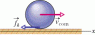# Question on Sliding/rolling spherical ball

• i_hate_math
In summary, a bowler throws a bowling ball of radius 11 cm with initial speed 7.8 m/s and initial angular speed 0 along a lane with coefficient of kinetic friction 0.13. The ball experiences linear acceleration of -1.274 m/s^2 and angular acceleration of 28.9545 rad/s^2 in the clockwise direction. It slides for 1.74927 seconds before stopping and rolling smoothly. The ball slides a distance that is not specified and has a linear speed of 6.551 m/s when smooth rolling begins.

## Homework Statement

A bowler throws a bowling ball of radius R = 11 cm along a lane. The ball (the figure) slides on the lane with initial speed vcom,0 = 7.8 m/s and initial angular speed ω0 = 0. The coefficient of kinetic friction between the ball and the lane is 0.13. The kinetic frictional force http://edugen.wileyplus.com/edugen/courses/crs7165/art/qb/qu/c11/low_fvec.gifkacting on the ball causes a linear acceleration of the ball while producing a torque that causes an angular acceleration of the ball. When speed vcom has decreased enough and angular speed φ has increased enough, the ball stops sliding and then rolls smoothly. During the sliding, what are the ball's (a) linear acceleration and (b) angular acceleration? (c) How long does the ball slide? (d) How far does the ball slide? (e) What is the linear speed of the ball when smooth rolling begins? Note that the clockwise direction is taken as negative.

## Homework Equations

Second Laws: F=m*a and. Torque=I*angular acceleration

I=(2/5)mR^2 ------ this I am not sure. This is probs where i went wrong.

## The Attempt at a Solution

For part a, I used Newtons second law
-m*a=friction force
thus a=-frictionconstant * 9.8=-1.274

Part b,
where i too I to be I=(2/5)mR^2
and i got α=28.9545
But this is WRONG

Part C i got 1.74927... which is correct
Part D is wrong followed by the wrong answer from part B
Part e i got the right answer

#### Attachments

•image.gif
2.7 KB · Views: 471
I agree with the magnitude of the angular acceleration you got for part b, but note the instruction that clockwise is to be taken as negative. Relate that to the picture.

•i_hate_math